# Vue - about computed (the problem of return function in calculated attribute)

2021-08-27 00:15:55 Mikey

I came across someone in the project computed Write code like this in ：

``````computed: {
getName() {
return function () {
return " Zhang San ";
};
},
},
Copy code ``````

There seems to be nothing wrong with this code , In fact, it violates computed Design intention of ,computed The reason for the design is to simplify the long and smelly calculation logic in the template , Make the template code look more concise , Easy to maintain , And the calculated properties are cached based on responsive dependencies , To optimize performance .

The official description of calculation properties is as follows ：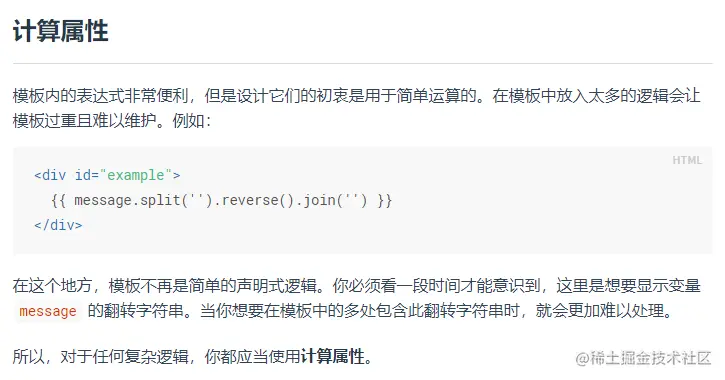Document address ： cn.vuejs.org/v2/guide/co…

Why does the above code violate computed The original intention of the design ？

First look at it. computed and methods The difference between , Then we can explain the problem , The official description is as follows ：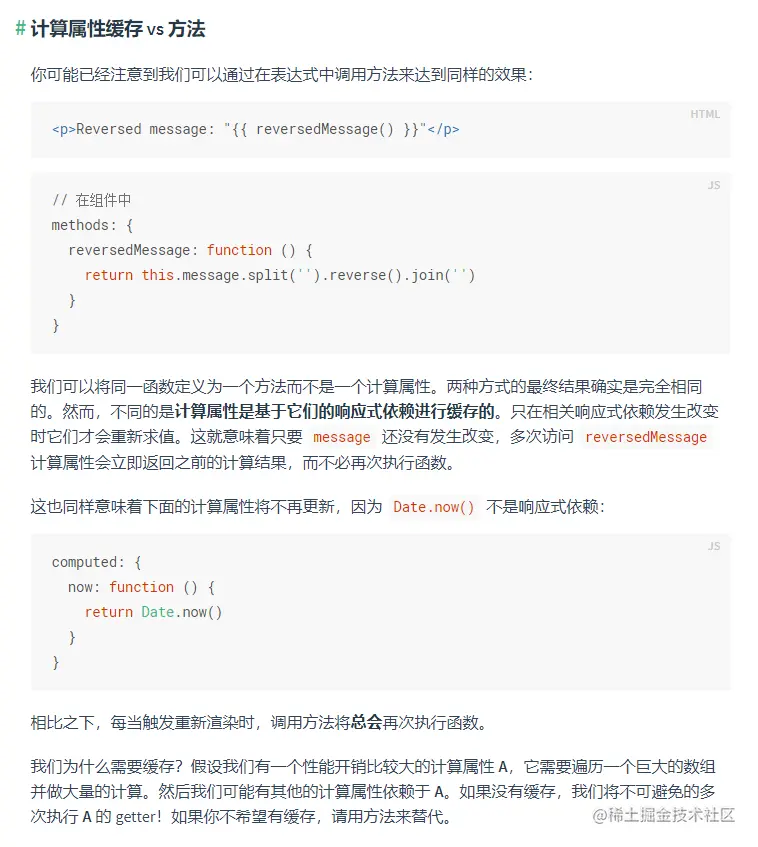In other words, although they have the same effect , but computed Cache based on responsive dependencies ,methods Can't , So let's verify that ：

First create computed and methods, Print log , Then call... Several times on the page

``````<template>
<div> <p>{{ computed_getName }}</p> <p>{{ computed_getName }}</p> <p>{{ computed_getName }}</p> <p>{{ computed_getName }}</p> <p>{{ method_getName() }}</p> <p>{{ method_getName() }}</p> <p>{{ method_getName() }}</p> <p>{{ method_getName() }}</p> </div>
</template>

<script> export default { computed: { computed_getName() { console.log("computed The calculated property is called "); return " Zhang San "; }, }, methods: { method_getName() { console.log("methods Method is called "); return " Li Si "; }, }, }; </script>
Copy code ``````

The page runs as follows ：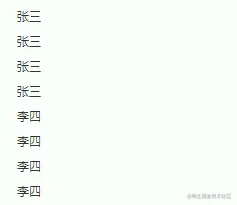The console output is as follows ：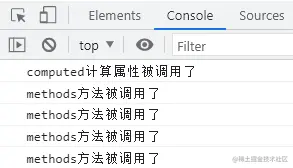As a result, you can see that although the calculated properties computed_getName With the method method_getName Are referenced in the template 4 Time , however computed_getName Only triggered 1 Time , and method_getName Method triggered 4 Time , That's what it's about computed Features with caching .

If you change the calculation attribute , Change to what you said at the beginning return Function? ？

``````<template>
<div> <!--  The corresponding call calculation attribute should also be changed to the form of function  --> <p>{{ computed_getName() }}</p> <p>{{ computed_getName() }}</p> <p>{{ computed_getName() }}</p> <p>{{ computed_getName() }}</p> <p>{{ method_getName() }}</p> <p>{{ method_getName() }}</p> <p>{{ method_getName() }}</p> <p>{{ method_getName() }}</p> </div>
</template>

<script> export default { computed: { computed_getName() { //  Modified into return Form of function  return function () { console.log("computed The calculated property is called "); return " Zhang San "; }; }, }, methods: { method_getName() { console.log("methods Method is called "); return " Li Si "; }, }, }; </script>
Copy code ``````

The page runs as follows ：The console output is as follows ：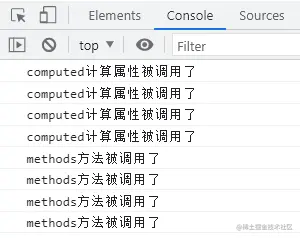The results are clear ,return After the function , To get the calculation result, you must call this calculation function （ Equivalent to calling a method , It's just that the location of the defined method is computed in ）, It will lose the function of cache , Another product Vue Official documents ：

Why do we need caching ？ Let's say we have a computing property with high performance overhead  A, It needs to traverse a huge array and do a lot of calculations . Then we may have other computational properties that depend on  A. If there is no cache , We will inevitably carry out many times  A  Of getter！ If you don't want a cache , Please use method instead of .

that , Suppose... In this example getName Is an expensive computational property , Now it has lost its cache function , Can we follow the official agreement to use methods instead ？

Another reason is , The creation steps of a calculated attribute are complex , If you are interested, please refer to the following articles for learning （ Compare the lazy , There are ready-made ones posted here , Light spray ...）：

cloud.tencent.com/developer/a…

The creation of a method is relatively simple , Source code is as follows ：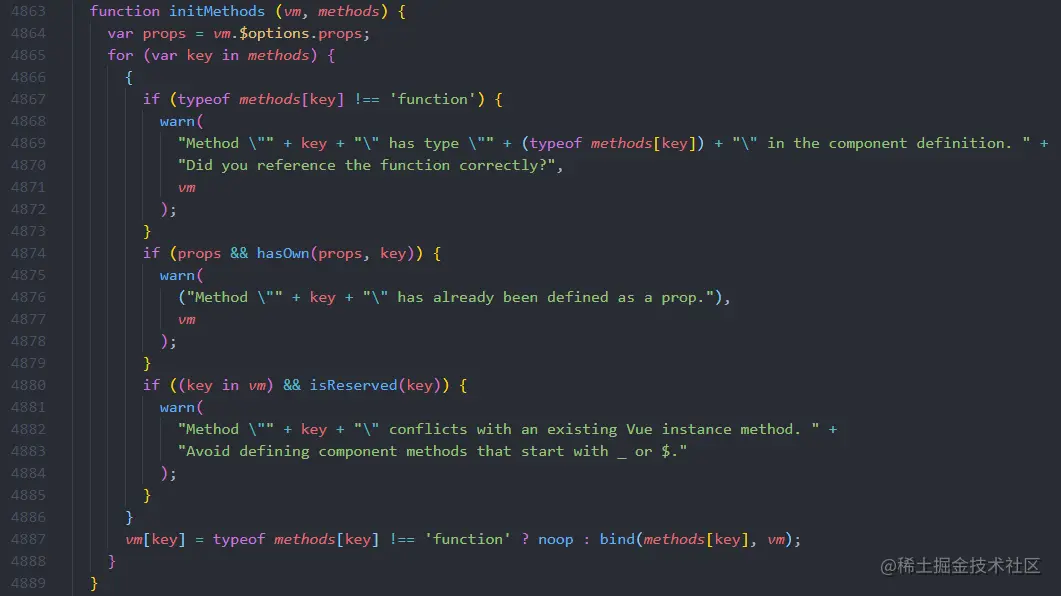Get rid of this pile of judgment conditions , In fact, it's just traversing all methods Then mount to the instance vm On .

therefore , If you see someone else computed in return The operation of the function , Do you know what to do ？